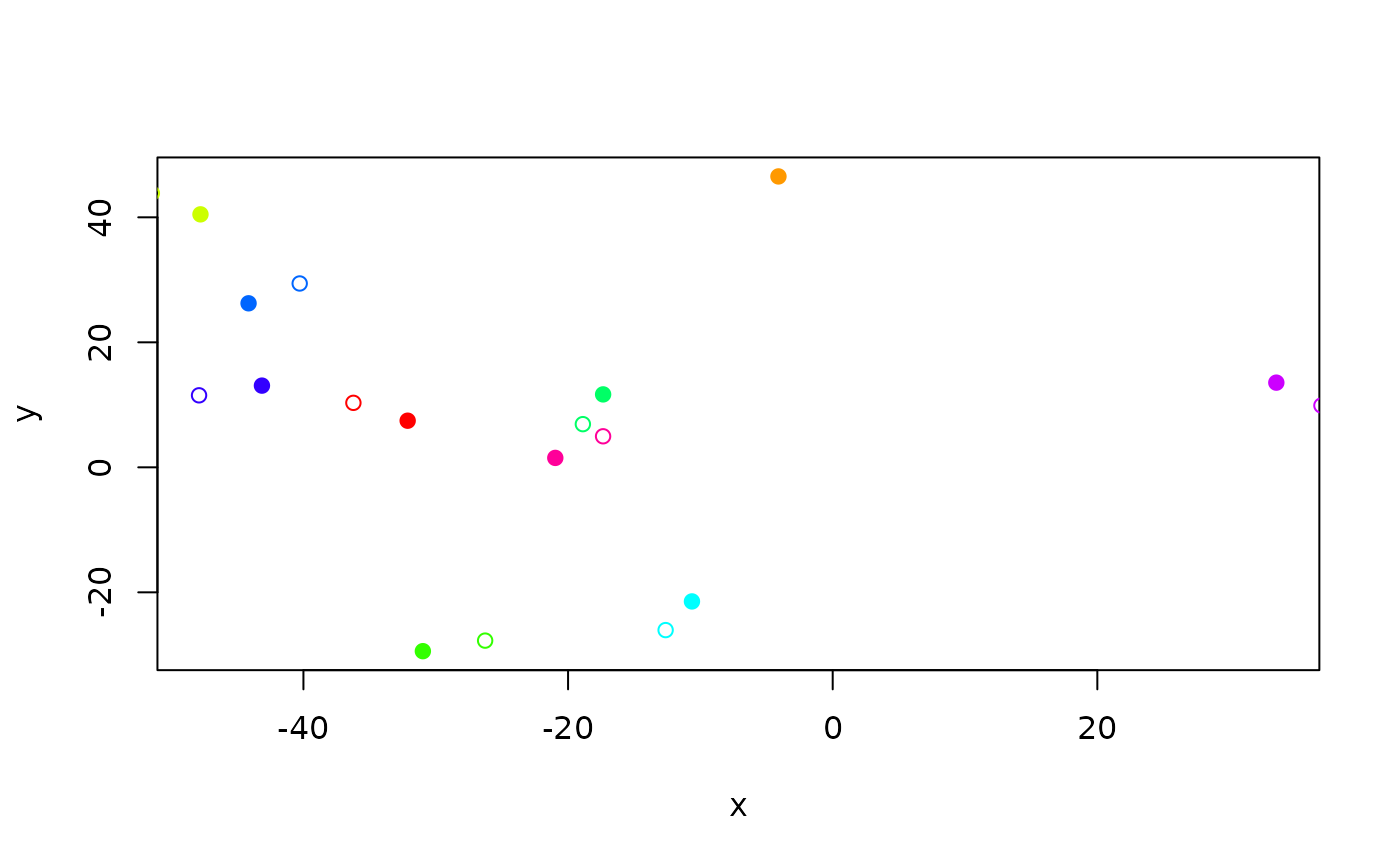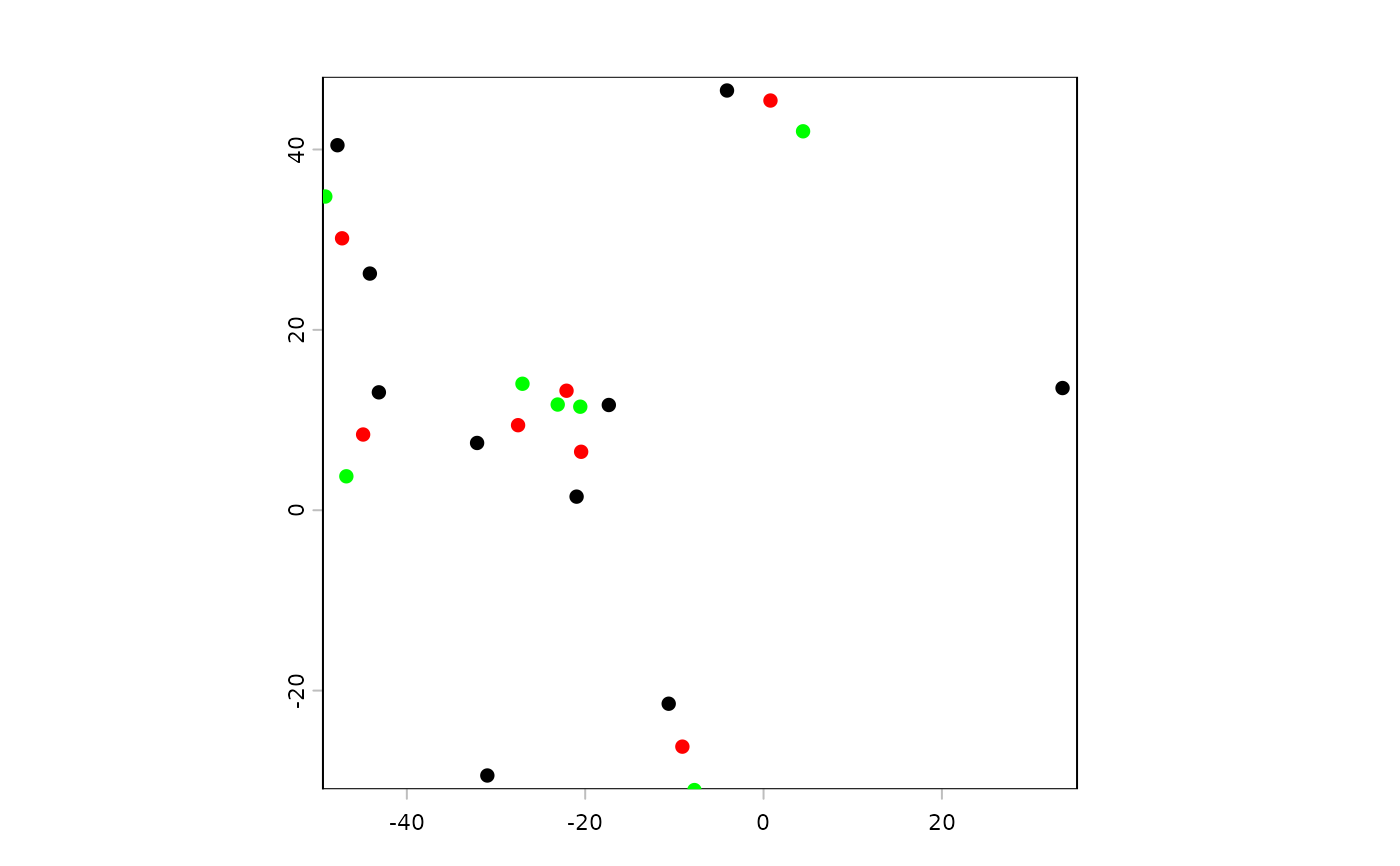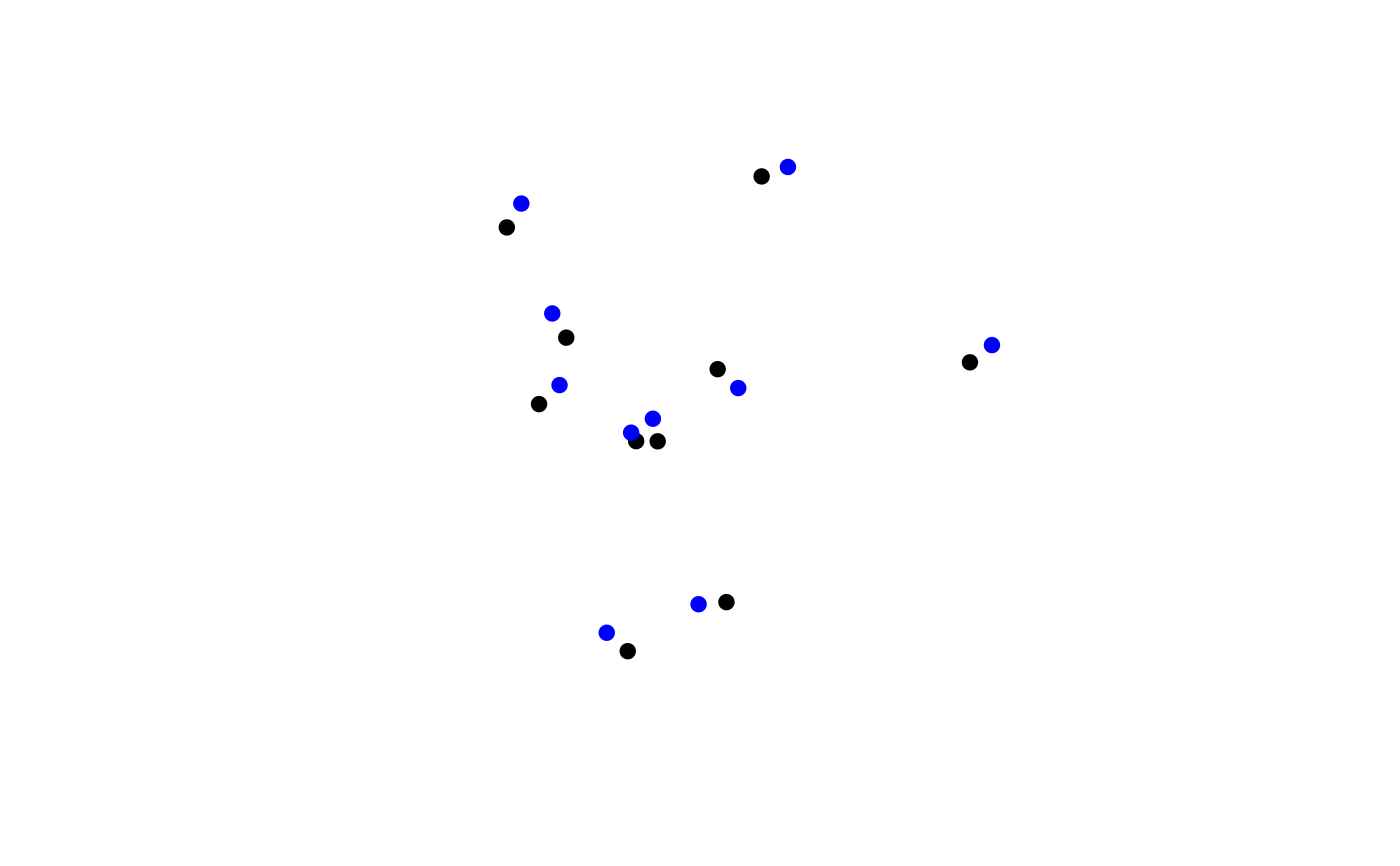Wrapper for selecting different animal movement methods.

This version uses just turn angles and step lengths to define the correlated random walk.

move(hypothesis = "crw", ...)

crw(
agent,
extent,
stepLength,
stddev,
lonlat = FALSE,
torus = FALSE,
returnMatrix = FALSE
)

## Arguments

hypothesis

Character vector, length one, indicating which movement hypothesis/method to test/use. Currently defaults to 'crw' (correlated random walk) using crw.

...

arguments passed to the function in hypothesis

agent

A SpatVector points geometry or a SpatialPoints* (deprecated) object. If is has attributes, e.g., SpatialPointsDataFrame, 2 of the columns must be x1 and y1, indicating the previous location. If it does not have these columns as attributes, x1 and y1 will be assigned randomly.

extent

An optional Extent object that will be used for torus.

stepLength

Numeric vector of length 1 or number of agents describing step length.

stddev

Numeric vector of length 1 or number of agents describing standard deviation of wrapped normal turn angles.

lonlat

Logical. If TRUE, coordinates should be in degrees. If FALSE coordinates represent planar ('Euclidean') space (e.g. units of meters)

torus

Logical. Should the movement be wrapped to the opposite side of the map, as determined by the extent argument. Default FALSE.

returnMatrix

If TRUE then the return object will be a matrix. This will be MUCH faster than retaining the sp or SpatVector class, and thus will be much more effective for iterative crw calls

## Value

A SpatVector points object with updated spatial position defined by a single occurrence of step length(s) and turn angle(s).

## Details

This simple version of a correlated random walk is largely the version that was presented in Turchin 1998, but it was also used with bias modifications in McIntire, Schultz, Crone 2007.

terra::distance()

wrap()

Eliot McIntire

## Examples

origDTThreads <- data.table::setDTthreads(2L)
origNcpus <- options(Ncpus = 2L)

# using just matrix
N <- 10
xrange <- yrange <- c(-50, 50)
starts <- cbind(x = stats::runif(N, xrange, xrange),
y = stats::runif(N, yrange, yrange))
moved <- crw(starts, stepLength = 5, stddev = 10)
plot(starts, col = rainbow(10), pch = 19)
points(moved, col = rainbow(10))# as SpatVector
agent <- terra::vect(starts)
moved <- crw(agent, stepLength = 5, stddev = 10)
movedAgain <- crw(moved, stepLength = 5, stddev = 10)
terra::plot(agent)
terra::plot(moved, add = TRUE, col = "red")
terra::plot(movedAgain, add = TRUE, col = "green")# 1000x faster!! -- returnMatrix = TRUE
agentOrig <- agent
reps <- 1e2
system.time({
for (i in 1:reps) agent <- crw(agent, stepLength = 5, stddev = 10, returnMatrix = TRUE)
})
#>    user  system elapsed
#>   0.007   0.000   0.007
agent <- agentOrig
system.time({
for (i in 1:reps) agent <- crw(agent, stepLength = 5, stddev = 10)
})
#>    user  system elapsed
#>     0.8     0.0     0.8

# as sp
if (requireNamespace("sp")) {
agent <- sp::SpatialPoints(starts)
spdf <- crw(agent, stepLength = 5, stddev = 10)
spdfNew <- crw(spdf, stepLength = 5, stddev = 10)
terra::plot(spdf, pch = 19)
terra::points(spdfNew, col = "blue", pch = 19)
}
#> agent does not have columns named x1 and y1, which represent the 'previous' locations. Assigning random values to those columns.
#> agent does not have columns named x1 and y1, which represent the 'previous' locations. Assigning random values to those columns.# clean up